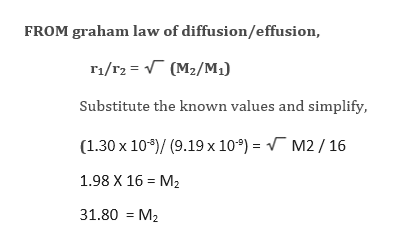# Methane, CH4, effuses through a small opening in the side of a container at a rate of 1.30 x 10-8 mol/s. An unknown gas effuses through the same opening at the rate of 9.19 x 10-9 mol/s when maintained at the same temperature as methane. Which of the following could be the unknown gas? a. O2 b. Xe c. Kr d. Ne e. H2

Question
191 views

Methane, CH4, effuses through a small opening in the side of a container at a rate of 1.30 x 10-8 mol/s. An unknown gas effuses through the same opening at the rate of 9.19 x 10-9 mol/s when maintained at the same temperature as methane. Which of the following could be the unknown gas?

a. O2 b. Xe c. Kr d. Ne e. H2

check_circle

Step 1

Given:

Rate of diffusion of Methane = 1.30 x 10-8 mol/s. = r1

Rate of diffusion of unknown = 9.19 x 10-9 mol/s= r2

Molar mass of Methane = 16 =M1

Molar mass of unknow = ? = M2

By calculating the molar mass of unknown we can identify the gas.

Step 2help_outlineImage TranscriptioncloseFROM graham law of diffusion/effusion, 1/r2 (M2/M,) Substitute the known values and simplify, (1.30 x 10)/ (9.19 x 10) = M2 /16 1.98 X 16 M2 31.80 M2 fullscreen

### Want to see the full answer?

See Solution

#### Want to see this answer and more?

Solutions are written by subject experts who are available 24/7. Questions are typically answered within 1 hour.*

See Solution
*Response times may vary by subject and question.
Tagged in

### Chemistry